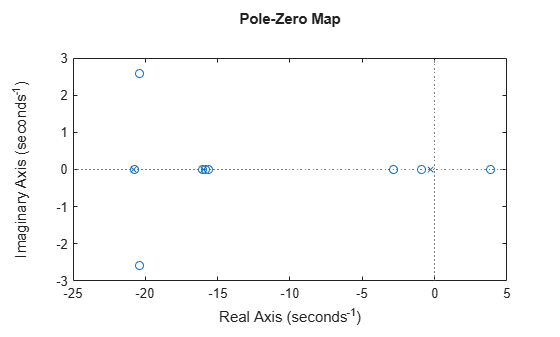Documentation

# iopzplot

Plot pole-zero map for I/O pairs and return plot handle

## Syntax

```h = iopzplot(sys) iopzplot(sys1,sys2,...) iopzplot(AX,...) iopzplot(..., plotoptions) ```

## Description

`h = iopzplot(sys)` computes and plots the poles and zeros of each input/output pair of the dynamic system model `sys`. The poles are plotted as x's and the zeros are plotted as o's. It also returns the plot handle h. You can use this handle to customize the plot with the `getoptions` and `setoptions` commands. Type

```help pzoptions ```

for a list of available plot options. For more information on the ways to change properties of your plots, see Ways to Customize Plots.

`iopzplot(sys1,sys2,...) `shows the poles and zeros of multiple dynamic system models `sys1,sys2,...` on a single plot. You can specify distinctive colors for each model, as in

```iopzplot(sys1,'r',sys2,'y',sys3,'g') ```

`iopzplot(AX,...)` plots into the axes with handle `AX`.

`iopzplot(..., plotoptions)` plots the poles and zeros with the options specified in `plotoptions`. Type

```help pzoptions ```

for more detail.

The function `sgrid` or `zgrid` can be used to plot lines of constant damping ratio and natural frequency in the s or z plane.

For arrays `sys` of LTI models, `iopzplot` plots the poles and zeros of each model in the array on the same diagram.

## Examples

collapse all

Create a pole/zero map of a two-input, two-output dynamic system.

```sys = rss(3,2,2); h = iopzplot(sys);```By default, the plot displays the poles and zeros of each I/O pair on its own axis. Use the plot handle to view all I/Os on a single axis.

`setoptions(h,'IOGrouping','all')`View the poles and zeros of a sixth-order state-space model estimated from input-output data. Use the plot handle to display the confidence intervals of the identified model's pole and zero locations.

```load iddata1 sys = ssest(z1,6,ssestOptions('focus','simulation')); h = iopzplot(sys); showConfidence(h)```There is at least one pair of complex-conjugate poles whose locations overlap with those of a complex zero, within the 1-σ confidence region. This suggests their redundancy. Hence, a lower (4th) order model might be more robust for the given data.

```sys2 = ssest(z1,4,ssestOptions('focus','simulation')); h = iopzplot(sys,sys2); showConfidence(h) legend('6th-order','4th-order') axis([-20, 10 -30 30])```The fourth-order model `sys2` shows less variability in the pole-zero locations.